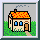## Geometry

is the first and - at school - the only topic to experience the force and function of logic deduction. It is not exhausted in noticing and learning the properties and anatomies of triangles and and conics. Its essence is the deduction of it all from a noticeably small set of suppositions, called axioms.

In connection with relativity and cosmology we learn to use the logical stategies of the familiar geometry to develop pseudo-euclidean and other non-euclidean geometries without other changes than in the supposition of the reflection procedure.

The pseudo-euclidean geometry takes from relativity the axiom that the speed of light does not change in compositions with the motion of a mirror. In geometric terms, we suppose two families of parallel straight lines (the registration traces of light signals) which are reflected onto each other and nothing else. To obtain the image of a point, take the two light-signal traces (called isotropic lines) through this point, reflect them at the mirror, and find the image in the intersection of the image lines.

Using this prescription for constructing reflections, the central triangle in the movie is a rectangular one, the right angle being the one at the point at rest. The movie shows a series of such rectangular triangles. The diamonds in green are squares in the new geometry, and their areas result in a² - b² = c².

The focal points of a conic lie now outside the conic, there are real tangents from the foci to the conic, and these tangents are isotropic lines. Conics play a fundamental role in the non-euclidean geometries: a favorite playground of mine.

As Jean-Baptiste le Rond d'Alembert wrote to Frederic II: La géométrie est une espèce de hochet que la nature nous a jeté pour nous consoler et nous amuser dans les ténèbres.

The leading topis are

the infinite,
the polarity between lines and points at a conic,
the focal points in non-euclidean geometry,

Figures and movies of different lectures are found starting with the projective-geometry chapter